true
true

Abhimanyu KumarChaukaghat, Varanasi, India - 221002# Abhimanyu Kumar## Mechanical engineer with 2 years of teaching exper...

Chaukaghat, Varanasi, India - 221002.

3 Students taught

5.0

UrbanPro Rating

Details verified of Abhimanyu Kumar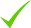IdentityEducation

Know how UrbanPro verifies Tutor details

Identity is verified based on matching the details uploaded by the Tutor with government databases.

## Overview

I am a engineer.i am giving home Tuition since last 2 years. I have a degree in mechanical engineering. my teaching skills is different from the other. I start from basic to advance level.
I am passionate about teaching. I can give a lot to the student. My aim is to always provide quality content

## Languages Spoken

Hindi Mother Tongue (Native)

English Proficient

## Education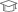Aktu 2018

Bachelor of Technology (B.Tech.)

Chaukaghat, Varanasi, India - 221002

## Verified Info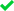ID VerifiedEducation VerifiedPhone VerifiedEmail VerifiedFacebook Verified

Report this Profile

Is this listing inaccurate or duplicate? Any other problem?

Type the letters as shown below *

Please enter the letters as show below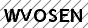Class 10 Tuition

Class LocationStudent's Home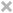Tutor's HomeOnline (video chat via skype, google hangout etc)

Years of Experience in Class 10 Tuition

2

Board

State, CBSE, ICSE

CBSE Subjects taught

Mathematics, Computer Practices, Science

ICSE Subjects taught

Mathematics, Physics

Taught in School or College

No

State Syllabus Subjects taught

Mathematics, Science

## Reviews (1)

this is test message this is test message this is test message this is test message this is test message this is test message this is test message

5.0 out of 5.0 1 review

Abhimanyu Kumar https://s3-ap-southeast-1.amazonaws.com/tv-prod/member/photo/6778184-small.jpg Chaukaghat
5.0051
Abhimanyu Kumar
A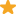"Best teacher you will get. His tips and tricks for concepts of physics are awesome. Great Teacher. Thank you very much for your support. Highly recommend. "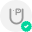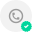Have you attended any class with Abhimanyu? Write a Review

## FAQs

1. Which school boards of Class 10 do you teach for?

State, CBSE and ICSE

2. Do you have any prior teaching experience?

No

3. Which classes do you teach?

I teach BSc Tuition, BTech Tuition, Class 10 Tuition, Class 11 Tuition and Class 12 Tuition Classes.

4. Do you provide a demo class?

Yes, I provide a free demo class.

5. How many years of experience do you have?

I have been teaching for 2 years.

Answered on 21/10/2019 CBSE/Class 12/Science/Physics/Unit 1-Electrostatics/ELECTROSTATIC POTENTIAL AND CAPACITANCE/NCERT Solutions/Exercise 2

We know, capacitance = dielectric constant × C C = KC = 6 × 18pF = 108pF (a) if the voltage supply remained connected , then the potential difference across the capacitor will remain the same. e.g., V = 100V and hence, charge on the capacitor becomes Q = CV = 108pF × 100VQ = 108 ×... ...more

We know, capacitance = dielectric constant × C₀
C = KC₀ = 6 × 18pF = 108pF [ see question - 2.8]
(a) if the voltage supply remained connected , then the potential difference across the capacitor will remain the same. e.g., V = 100V and hence, charge on the capacitor becomes Q = CV = 108pF × 100V
Q = 108 × 10⁻¹² × 100 = 1.08 × 10⁻⁸ C

(b) if the voltage supply was disconnected , then charge on the capacitor reamins the same. e.g., Q = 1.8 × 10⁻⁹C [ see question - 2.8]
And hence the potential difference across the capacitor becomes
V = Q/C = 1.8 × 10⁻⁹/1.08 × 10⁻¹⁰ = 16.6V

Dislike Bookmark

Answered on 18/10/2019 CBSE/Class 12/Science/Physics/Unit 1-Electrostatics/ELECTROSTATIC POTENTIAL AND CAPACITANCE/NCERT Solutions/Exercise 2

A regular hexagon ABCDEF of side 10cm has a charge 5 μC at each of its vertices as shown in the figure.Potential due to charge placed at A, at the centre is Kq/rPotential due to charge placed at B, at the centre is Kq/rPotential due to Charge placed at F , at centre is Kq/rso, total potential at centre... ...more

A regular hexagon ABCDEF of side 10cm has a charge 5 μC at each of its vertices as shown in the figure.
Potential due to charge placed at A, at the centre is Kq/r
Potential due to charge placed at B, at the centre is Kq/r

Potential due to Charge placed at F , at centre is Kq/r
so, total potential at centre of regular hexagon , V = V₁ + V₂ + V₃ + V₄ + V₅ + V₆
so, V = kq/r + kq/r + kq/r + kq/r + kq/r + Kq/r
V = 6kq/r
Hence, k = 9 × 10⁹Nm²/C² , q = 5 × 10⁻⁶C and r = 10cm = 0.1 m
Now, V = 6 × 9 × 10⁹ × 5 × 10⁻⁶/0.1 = 2.7 × 10⁶ Volts.

Dislike Bookmark

Answered on 18/10/2019 CBSE/Class 12/Science/Physics/Unit 1-Electrostatics/ELECTROSTATIC POTENTIAL AND CAPACITANCE/NCERT Solutions/Exercise 2

initially d, therefore, C= EA/d=8 -(1) now d1=d/2, and dielectric is put, therefore C1=KEA/d1. -(2) here k=6 which is dielectric constant we know d1=d/2 substituting it in (1) C1=6EA/(d/2)=12EA/d. -(3) (3)÷(1) C1÷8=12EA/d÷EA/d C1=96 uF ...more

initially d,

therefore, C= EA/d=8 -(1)

now d1=d/2, and dielectric is put,

therefore C1=KEA/d1. -(2) here k=6 which is dielectric constant

we know d1=d/2 substituting it in (1)

C1=6EA/(d/2)=12EA/d. -(3)

(3)÷(1)

C1÷8=12EA/d÷EA/d

C1=96 uF

Dislike Bookmark

Answered on 18/10/2019 CBSE/Class 12/Science/Physics/Unit 1-Electrostatics/ELECTROSTATIC POTENTIAL AND CAPACITANCE/NCERT Solutions/Exercise 2

a) We know, charge have nature to reside outer surface of the conductor. It means, charge inside the surface equals zero.According to Gaussian theorem, = q/ε , here q is charged inclosed the Gaussian surface. q = 0so, = 0 and flux , = E.A = 0so, E = 0Hence, inside the sphere, the electric... ...more

a) We know, charge have nature to reside outer surface of the conductor. It means, charge inside the surface equals zero.
According to Gaussian theorem,
Ф = q/ε ₀, here q is charged inclosed the Gaussian surface.
∵ q = 0
so, Ф = 0 and flux , Ф = E.A = 0
so, E = 0
Hence, inside the sphere, the electric field equals zero.
(b) Take a Gaussian surface of radius r > R = 12cm
then, charged inclosed into the Gaussian surface is q = 1.6 × 10⁻⁷ C
So, Ф = q/ε₀
So, EA = q/ε₀
E = q/ε₀A, here A is the surface area of Gaussian spherical surface
e.g., A = 4πr²
So, E = q/4πε₀r² = 9 × 10⁹ × 1.6 × 10⁻⁷/(12 × 10⁻²)²
= 10⁵ N/C
(C) Similarly explanation of (B),
So, E = kq/r²
Here , k = 9 × 10⁹ Nm²/C² , q = 1.6 × 10⁻⁷C and r = 18cm
So, E = 9 × 10⁹ × 1.6 × 10⁻⁷/(18 × 10⁻²)²
= 4.44 × 10⁴ N/C.

Dislike Bookmark

Answered on 18/10/2019 CBSE/Class 12/Science/Physics/Unit 1-Electrostatics/ELECTROSTATIC POTENTIAL AND CAPACITANCE/NCERT Solutions/Exercise 2

(a) an equipotential surface is a plane on which potential is zero everywhere.This plane is normal to the plane in which two point charges are placed.Let normal plane be placed at C of x distance from +2μC charge.Then, potential due to 2μC + potential due to -2μC = 0Kq/x + k(-q)/(6 - x) = 0x... ...more

(a) an equipotential surface is a plane on which potential is zero everywhere.
This plane is normal to the plane in which two point charges are placed.
Let normal plane be placed at C of x distance from +2μC charge.
Then, potential due to 2μC + potential due to -2μC = 0
Kq/x + k(-q)/(6 - x) = 0
x = 3 cm
Hence, the equipotential surface of the system appears at midpoint of the system of two given charges, and it is normal to the plane of charges.
(B) we know, the direction of the electric field from positive to negative charge.
So, the direction of the electric field at every point on the surface is normal to the plane in the direction AB.

Dislike Bookmark
Class 10 Tuition 5.0

Class LocationStudent's HomeTutor's HomeOnline (video chat via skype, google hangout etc)

Years of Experience in Class 10 Tuition

2

Board

State, CBSE, ICSE

CBSE Subjects taught

Mathematics, Computer Practices, Science

ICSE Subjects taught

Mathematics, Physics

Taught in School or College

No

State Syllabus Subjects taught

Mathematics, Science

Class 11 Tuition 5.0

Class LocationStudent's HomeTutor's HomeOnline (video chat via skype, google hangout etc)

Years of Experience in Class 11 Tuition

1

Board

State, ISC/ICSE, CBSE

ISC/ICSE Subjects taught

Computer Science, Mathematics, Physics

CBSE Subjects taught

Physics, Mathematics, Computer Science

Taught in School or College

No

State Syllabus Subjects taught

Mathematics, Physics, Computer Science

Class 12 Tuition 5.0

Class LocationStudent's HomeTutor's HomeOnline (video chat via skype, google hangout etc)

Years of Experience in Class 12 Tuition

2

Board

State, ISC/ICSE, CBSE

ISC/ICSE Subjects taught

Mathematics, Physics

CBSE Subjects taught

Physics, Mathematics

Taught in School or College

No

State Syllabus Subjects taught

Mathematics, Physics

BTech Tuition 5.0

Class LocationStudent's HomeTutor's HomeOnline (video chat via skype, google hangout etc)

Years of Experience in BTech Tuition

1

BTech Mechanical subjects

Manufacturing Technology, Welding Technology, Composites: Mechanics & Processing, Mechanics of Machines, Fluid Mechanics, Operations Research, Internal Combustion Engines and Emissions, Destructive & Non Destructive Testing/ Fracture Mechanics, Thermodynamics, Heat & Mass Transfer, Analysis and Design of Machine Components, Kinematics of Machinery, Machine Design, Material Science and Metallurgy, Strength of Materials

BTech Branch

BTech Mechanical Engineering

Type of class

Regular Classes, Crash Course

Class strength catered to

Group Classes, One on one/ Private Tutions

Taught in School or College

No

BSc Tuition 5.0

Class LocationStudent's HomeTutor's HomeOnline (video chat via skype, google hangout etc)

Years of Experience in BSc Tuition

2

BSc Physics Subjects

Mathematical Physics, Mechanics, Solid State Physics, Nuclear and Particle Physics, Statistical Physics, Mathematics, Optics, Numerical Analysis, Thermal Physics, Quantum Mechanics, Atomic and Molecular Physics, Electricity and Magnetism

BSc Computer Science Subjects

Operational Research

Type of class

Regular Classes, Crash Course

Class strength catered to

Group Classes, One on one/ Private Tutions

Taught in School or College

No

BSc Branch

BSc Physics, BSc Mathematics, BSc Computer Science

BSc Mathematics Subjects

Calculus, Differential Equations and Mathematical Modelling, Mechanics, Numerical Methods and Programming, Algebra, Probability and Statistics, Number Theory, Physics

this is test message this is test message this is test message this is test message this is test message this is test message this is test message

5.0 out of 5.0 1 review

Abhimanyu Kumar
A"Best teacher you will get. His tips and tricks for concepts of physics are awesome. Great Teacher. Thank you very much for your support. Highly recommend. "Have you attended any class with Abhimanyu? Write a Review

Answered on 21/10/2019 CBSE/Class 12/Science/Physics/Unit 1-Electrostatics/ELECTROSTATIC POTENTIAL AND CAPACITANCE/NCERT Solutions/Exercise 2

We know, capacitance = dielectric constant × C C = KC = 6 × 18pF = 108pF (a) if the voltage supply remained connected , then the potential difference across the capacitor will remain the same. e.g., V = 100V and hence, charge on the capacitor becomes Q = CV = 108pF × 100VQ = 108 ×... ...more

We know, capacitance = dielectric constant × C₀
C = KC₀ = 6 × 18pF = 108pF [ see question - 2.8]
(a) if the voltage supply remained connected , then the potential difference across the capacitor will remain the same. e.g., V = 100V and hence, charge on the capacitor becomes Q = CV = 108pF × 100V
Q = 108 × 10⁻¹² × 100 = 1.08 × 10⁻⁸ C

(b) if the voltage supply was disconnected , then charge on the capacitor reamins the same. e.g., Q = 1.8 × 10⁻⁹C [ see question - 2.8]
And hence the potential difference across the capacitor becomes
V = Q/C = 1.8 × 10⁻⁹/1.08 × 10⁻¹⁰ = 16.6V

Dislike Bookmark

Answered on 18/10/2019 CBSE/Class 12/Science/Physics/Unit 1-Electrostatics/ELECTROSTATIC POTENTIAL AND CAPACITANCE/NCERT Solutions/Exercise 2

A regular hexagon ABCDEF of side 10cm has a charge 5 μC at each of its vertices as shown in the figure.Potential due to charge placed at A, at the centre is Kq/rPotential due to charge placed at B, at the centre is Kq/rPotential due to Charge placed at F , at centre is Kq/rso, total potential at centre... ...more

A regular hexagon ABCDEF of side 10cm has a charge 5 μC at each of its vertices as shown in the figure.
Potential due to charge placed at A, at the centre is Kq/r
Potential due to charge placed at B, at the centre is Kq/r

Potential due to Charge placed at F , at centre is Kq/r
so, total potential at centre of regular hexagon , V = V₁ + V₂ + V₃ + V₄ + V₅ + V₆
so, V = kq/r + kq/r + kq/r + kq/r + kq/r + Kq/r
V = 6kq/r
Hence, k = 9 × 10⁹Nm²/C² , q = 5 × 10⁻⁶C and r = 10cm = 0.1 m
Now, V = 6 × 9 × 10⁹ × 5 × 10⁻⁶/0.1 = 2.7 × 10⁶ Volts.

Dislike Bookmark

Answered on 18/10/2019 CBSE/Class 12/Science/Physics/Unit 1-Electrostatics/ELECTROSTATIC POTENTIAL AND CAPACITANCE/NCERT Solutions/Exercise 2

initially d, therefore, C= EA/d=8 -(1) now d1=d/2, and dielectric is put, therefore C1=KEA/d1. -(2) here k=6 which is dielectric constant we know d1=d/2 substituting it in (1) C1=6EA/(d/2)=12EA/d. -(3) (3)÷(1) C1÷8=12EA/d÷EA/d C1=96 uF ...more

initially d,

therefore, C= EA/d=8 -(1)

now d1=d/2, and dielectric is put,

therefore C1=KEA/d1. -(2) here k=6 which is dielectric constant

we know d1=d/2 substituting it in (1)

C1=6EA/(d/2)=12EA/d. -(3)

(3)÷(1)

C1÷8=12EA/d÷EA/d

C1=96 uF

Dislike Bookmark

Answered on 18/10/2019 CBSE/Class 12/Science/Physics/Unit 1-Electrostatics/ELECTROSTATIC POTENTIAL AND CAPACITANCE/NCERT Solutions/Exercise 2

a) We know, charge have nature to reside outer surface of the conductor. It means, charge inside the surface equals zero.According to Gaussian theorem, = q/ε , here q is charged inclosed the Gaussian surface. q = 0so, = 0 and flux , = E.A = 0so, E = 0Hence, inside the sphere, the electric... ...more

a) We know, charge have nature to reside outer surface of the conductor. It means, charge inside the surface equals zero.
According to Gaussian theorem,
Ф = q/ε ₀, here q is charged inclosed the Gaussian surface.
∵ q = 0
so, Ф = 0 and flux , Ф = E.A = 0
so, E = 0
Hence, inside the sphere, the electric field equals zero.
(b) Take a Gaussian surface of radius r > R = 12cm
then, charged inclosed into the Gaussian surface is q = 1.6 × 10⁻⁷ C
So, Ф = q/ε₀
So, EA = q/ε₀
E = q/ε₀A, here A is the surface area of Gaussian spherical surface
e.g., A = 4πr²
So, E = q/4πε₀r² = 9 × 10⁹ × 1.6 × 10⁻⁷/(12 × 10⁻²)²
= 10⁵ N/C
(C) Similarly explanation of (B),
So, E = kq/r²
Here , k = 9 × 10⁹ Nm²/C² , q = 1.6 × 10⁻⁷C and r = 18cm
So, E = 9 × 10⁹ × 1.6 × 10⁻⁷/(18 × 10⁻²)²
= 4.44 × 10⁴ N/C.

Dislike Bookmark

Answered on 18/10/2019 CBSE/Class 12/Science/Physics/Unit 1-Electrostatics/ELECTROSTATIC POTENTIAL AND CAPACITANCE/NCERT Solutions/Exercise 2

(a) an equipotential surface is a plane on which potential is zero everywhere.This plane is normal to the plane in which two point charges are placed.Let normal plane be placed at C of x distance from +2μC charge.Then, potential due to 2μC + potential due to -2μC = 0Kq/x + k(-q)/(6 - x) = 0x... ...more

(a) an equipotential surface is a plane on which potential is zero everywhere.
This plane is normal to the plane in which two point charges are placed.
Let normal plane be placed at C of x distance from +2μC charge.
Then, potential due to 2μC + potential due to -2μC = 0
Kq/x + k(-q)/(6 - x) = 0
x = 3 cm
Hence, the equipotential surface of the system appears at midpoint of the system of two given charges, and it is normal to the plane of charges.
(B) we know, the direction of the electric field from positive to negative charge.
So, the direction of the electric field at every point on the surface is normal to the plane in the direction AB.

Dislike Bookmark

Abhimanyu Kumar describes himself as Mechanical engineer with 2 years of teaching experience. He conducts classes in BSc Tuition, BTech Tuition and Class 10 Tuition. Abhimanyu is located in Chaukaghat, Varanasi. Abhimanyu takes at students Home. He has 2 years of teaching experience . Abhimanyu has completed Bachelor of Technology (B.Tech.) from Aktu in 2018. He is well versed in English and Hindi. Abhimanyu has got 1 reviews till now with 100% positive feedback.

•• Want to learn from Abhimanyu?

• Contact Now
X

Recommended Profiles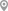Bazardiha, VaranasiNaibasti, VaranasiBaragaon (Varanasi), VaranasiBabatpur, VaranasiBulanala, VaranasiBhelupur, Varanasi

X

Let us shortlist and give the best tutors and institutes.

or

Send Enquiry to Abhimanyu

Let Abhimanyu know you are interested in their class